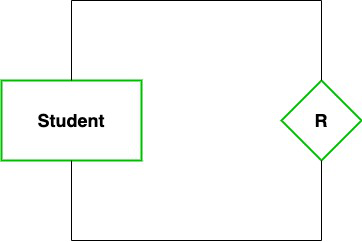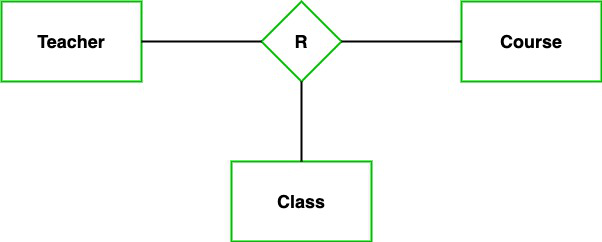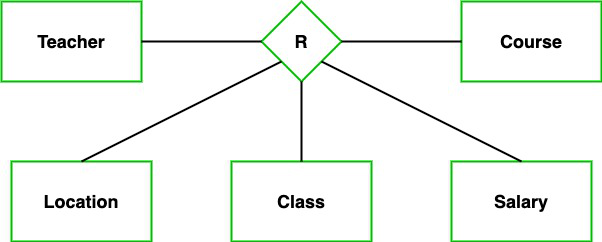GFG App
Open AppBrowser
Continue

# Degree of Relations in DBMS

We are living in a world where every entity has relationships with one other whether a living or non-living being. For example, you are a single entity but you share different relations with your family, friends. Even within a family-like, you are the son of your father at the same time you are also a sibling of your brother. Similarly the relationships exist in Database Management (DBMS). In this article, we are going to learn about what is the degree of relationships, and the types of relationships.

### Degree of Relationship

In DBMS, a degree of relationship represents the number of entity types that associate in a relationship. For example, we have two entities, one is a student and the other is a bag and they are connected with the primary key and foreign key. So, here we can see that the degree of relationship is 2 as 2 entities are associating in a relationship.

Types of degree

Now, based on the number of linked entity types, we have 4 types of degrees of relationships.

1. Unary
2. Binary
3. Ternary
4. N-ary

Let’s discuss them one by one with the help of examples.

### Unary

In this type of relationship, both the associating entity type are the same. So, we can say that unary relationships exist when both entity types are the same and we call them the degree of relationship is 1. Or in other words, in a relation only one entity set is participating then such type of relationship is known as a unary relationship.

Example: In a particular class, we have many students, there are monitors too. So, here class monitors are also students. Thus, we can say that only students are participating here. So the degree of such type of relationship is 1.### Binary (degree 2)

In a Binary relationship, there are two types of entity associates. So, we can say that a Binary relationship exists when there are two types of entity and we call them a degree of relationship is 2. Or in other words, in a relation when two entity sets are participating then such type of relationship is known as a binary relationship. This is the most used relationship and one can easily be converted into a relational table.

Example: We have two entity types ‘Student’ and ‘ID’ where each ‘Student’ has his ‘ID’. So, here two entity types are associating we can say it is a binary relationship. Also, one ‘Father’ can have many ‘daughters’ but each ‘daughter’ should belong to only one ‘father. We can say that it is a one-to-many binary relationship.### Ternary(degree 3)

In the Ternary relationship, there are three types of entity associates. So, we can say that a Ternary relationship exists when there are three types of entity and we call them a degree of relationship is 3. Since the number of entities increases due to this, it becomes very complex to turn E-R into a relational table. Now let’s understand with the examples.

Example: We have three entity types ‘Teacher’, ‘Course’, and ‘Class’. The relationship between these entities is defined as the teacher teaching a particular course, also the teacher teaches a particular class. So, here three entity types are associating we can say it is a ternary relationship.### N-ary (n degree)

In the N-ary relationship, there are n types of entity that associates. So, we can say that an N-ary relationship exists when there are n types of entities. There is one limitation of the N-ary relationship, as there are many entities so it is very hard to convert into an entity, rational table. So, this is very uncommon, unlike binary which is very much popular.

Example: We have 5 entities Teacher, Class, Location, Salary, Course. So, here five entity types are associating we can say an n-ary relationship is 5.My Personal Notes arrow_drop_up